# I Spy a Number M 3 N 1

• Slides: 9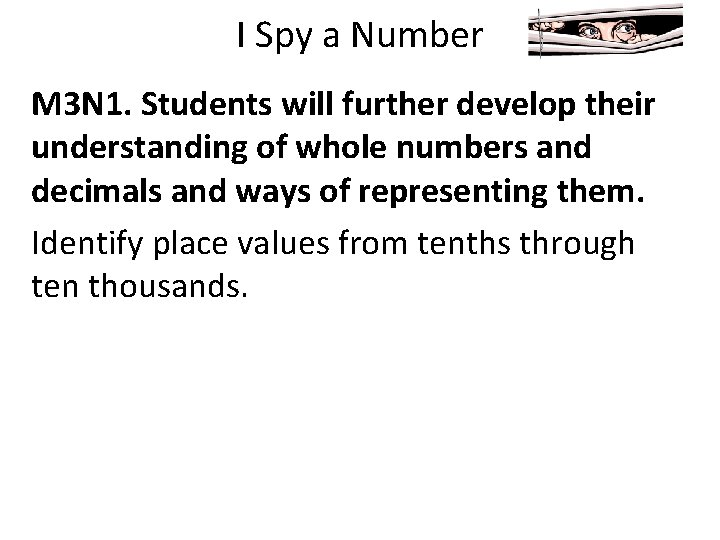I Spy a Number M 3 N 1. Students will further develop their understanding of whole numbers and decimals and ways of representing them. Identify place values from tenths through ten thousands.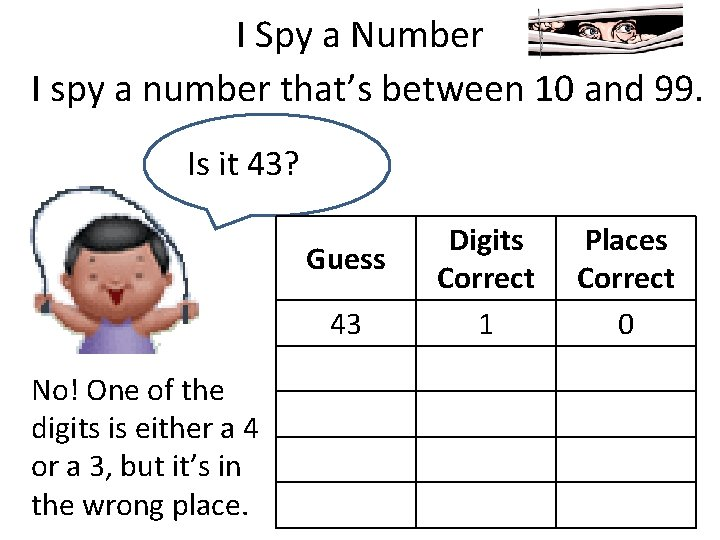I Spy a Number I spy a number that’s between 10 and 99. Is it 43? Guess 43 No! One of the digits is either a 4 or a 3, but it’s in the wrong place. Digits Correct 1 Places Correct 0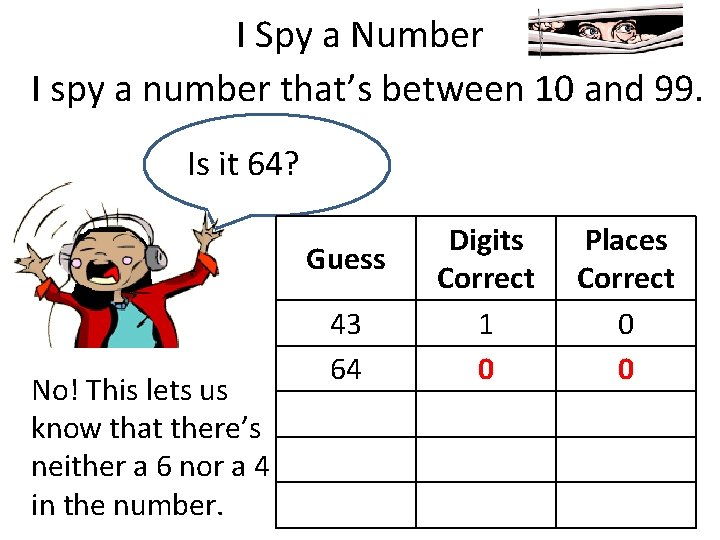I Spy a Number I spy a number that’s between 10 and 99. Is it 64? Guess No! This lets us know that there’s neither a 6 nor a 4 in the number. 43 64 Digits Correct 1 0 Places Correct 0 0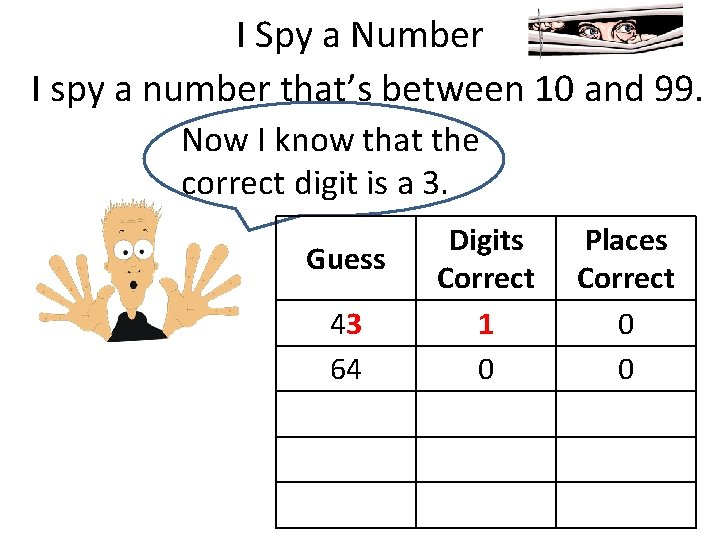I Spy a Number I spy a number that’s between 10 and 99. Now I know that the correct digit is a 3. Guess 43 64 Digits Correct 1 0 Places Correct 0 0I Spy a Number I spy a number that’s between 10 and 99. The 3 must be in the tens place. Guess 43 64 Digits Correct 1 0 Places Correct 0 0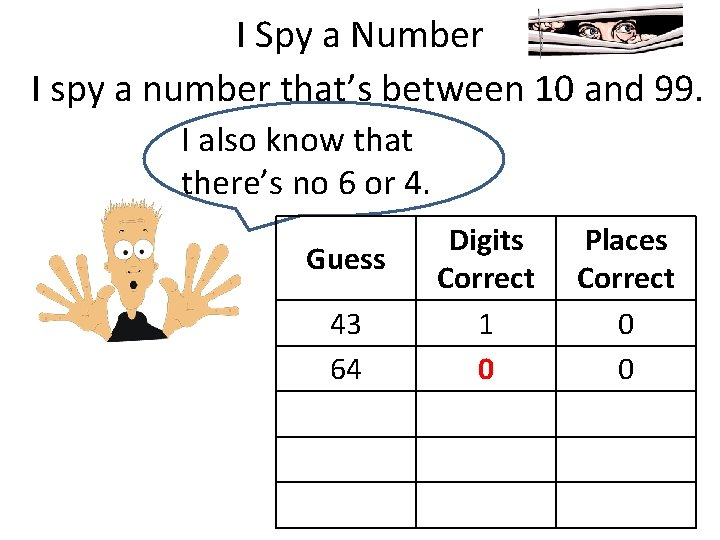I Spy a Number I spy a number that’s between 10 and 99. I also know that there’s no 6 or 4. Guess 43 64 Digits Correct 1 0 Places Correct 0 0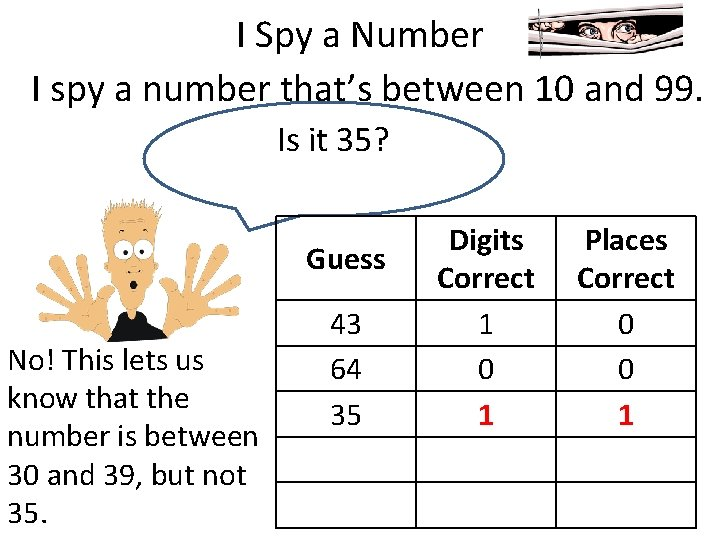I Spy a Number I spy a number that’s between 10 and 99. Is it 35? Guess No! This lets us know that the number is between 30 and 39, but not 35. 43 64 35 Digits Correct 1 0 1 Places Correct 0 0 1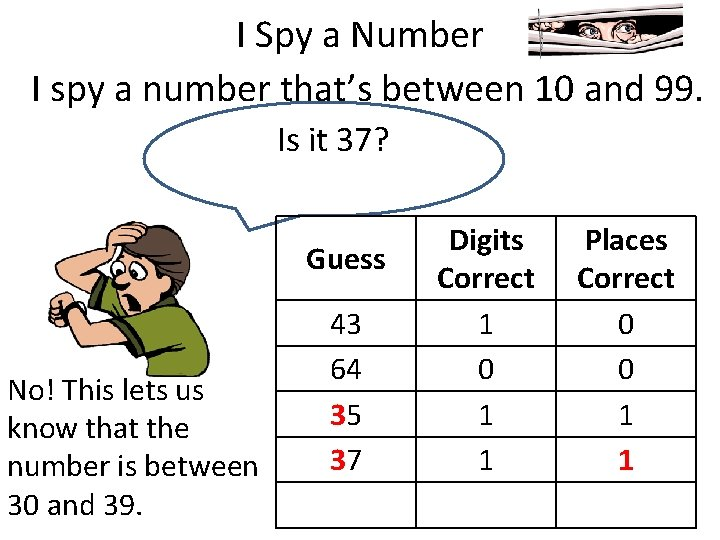I Spy a Number I spy a number that’s between 10 and 99. Is it 37? Guess No! This lets us know that the number is between 30 and 39. 43 64 35 37 Digits Correct 1 0 1 1 Places Correct 0 0 1 1I Spy a Number I spy a number that’s between 10 and 99. Is it 39? Guess Yes!! 43 64 35 37 39 Digits Correct 1 0 1 1 2 Places Correct 0 0 1 1 2